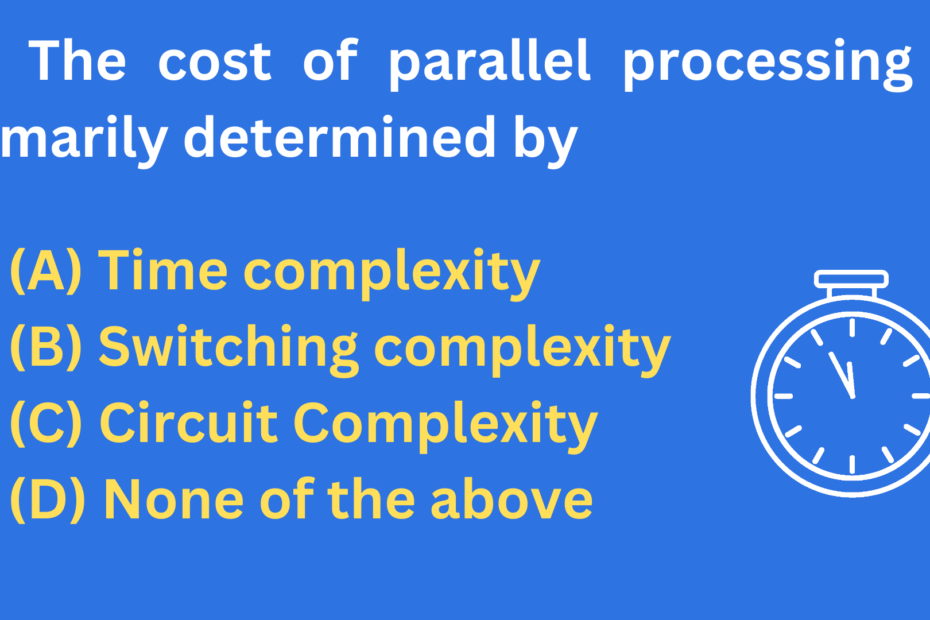# The cost of parallel processing is primarily determined by

• CS PYQ## The cost of parallel processing is primarily determined by

• (A) Time complexity
• (B) Switching complexity
• (C) Circuit Complexity
• (D) None of the above

## Parallel processing

• Parallel processing is the use of multiple processors or computers working together to complete a task.
• It allows tasks to be divided into smaller pieces and completed simultaneously, which can greatly increase the speed and efficiency of the task.
• Parallel processing is often used in scientific and mathematical computing, as well as in high-performance computing applications such as data analysis and machine learning.
• It can also be used to improve the performance of everyday tasks such as web browsing, video rendering, and online gaming.

## Time complexity

• Time complexity refers to the amount of time it takes for an algorithm or program to complete its task, typically measured in terms of the size of the input.
• For example, a time complexity of O(n) means that the time it takes for the algorithm to complete increases linearly with the size of the input (n). A time complexity of O(n^2) means that the time it takes increases at a rate of n squared, and so on.
• In general, the goal is to design algorithms with as low a time complexity as possible, as this will allow them to run faster and more efficiently.

## Switching complexity

• Switching complexity refers to the level of difficulty or effort required to switch between different tasks or activities.
• This can be affected by various factors such as the complexity of the tasks themselves, the amount of information or resources needed to complete them, and the amount of time and effort required to switch between them.
• High switching complexity can lead to reduced productivity and increased stress, as individuals may struggle to manage multiple tasks simultaneously or may experience difficulty adapting to new tasks or environments.
• On the other hand, low switching complexity can help individuals more easily and efficiently transition between tasks, leading to increased productivity and reduced stress.

## Circuit Complexity

• In computer science, circuit complexity is the study of the size of Boolean circuits, which are used to compute Boolean functions.
• A Boolean circuit is a directed acyclic graph, where the vertices are gates that perform Boolean operations, and the edges represent the flow of information.
• The size of a Boolean circuit is the number of gates it contains.
• The goal of circuit complexity is to determine how many gates are needed to compute a given function, and to understand the computational power of different types of circuits.
• Circuit complexity has applications in the design and analysis of algorithms, as well as in the study of computational complexity theory.

The cost of parallel processing is primarily determined by Switching complexity